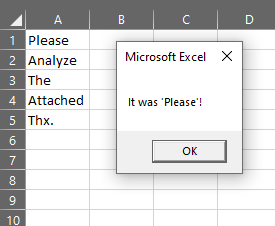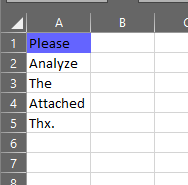# How to work with IF statements in VBA?

If Statements are logical alternatives that will execute something based on something. Theyare very intuitive as you are already doing them everyday in your

life, it is just a matter of learning the syntax for them (code language for saying how to write them in VBA).

For example, should I get coffee? if yes, then add cream, if not, get tea with honey.

### IF statements follows the following structure

```If Logical statement Then
'Do something if the logical statement is true.
End If```

## Examples of more IF statements

### Simple IF statement

```Sub If_One()
'One If condition

End If

End Sub```### IF statement with two conditions

```Sub If_Two()
'Two conditions
'as the first statement was incorrect.

ElseIf Range("A2") = "Analyze" Then
MsgBox ("It was 'Analyze'!")
End If

End Sub```### IF statement with more conditions

```Sub If_Mult()
​
'Multiple conditions
'Colors cell with 'Please' as the first if statement was correct.
'since the first IF statement was correct the code is done, and does
not check the other IFs.

Range("A1").Interior.Color = RGB(150, 200, 180) 'colors green

ElseIf Range("A2") = "Analyze" Then

Range("A2").Interior.Color = RGB(100, 100, 255) 'colors blue

ElseIf Range("A3") = "The" Then

Range("A3").Interior.Color = RGB(250, 180, 180) 'colors salmon

End If
​
End Sub```### IF statement with more conditions

```Sub If_Mult_Blue()

'Multiple conditions
'Colors cell with 'Please' blue according to the second IF statement.

If Range("A1") = "Analyze" Then

Range("A1").Interior.Color = RGB(150, 200, 180) 'colors green
Else
Range("A1").Interior.Color = RGB(100, 100, 255) 'colors blue

End If

End Sub```